# PSAT Math : How to find an angle in a hexagon

## Example Questions

200

170

210

180

190

### Example Question #2 : How To Find An Angle In A Hexagon

If a triangle has 180 degrees, what is the sum of the interior angles of a regular octagon?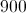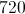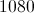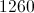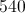Explanation:

The sum of the interior angles of a polygon is given by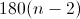where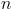= number of sides of the polygon.  An octagon has 8 sides, so the formula becomes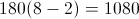### Example Question #2 : How To Find An Angle In A Hexagon

In a rectangular hexagon, what is the meaure of each interior angle?

105 degrees

90 degrees

72 degrees

120 degrees

150 degrees

120 degrees

Explanation:

The sum of the interior angles of a hexagon must equal 720 degrees. Because the hexagon is regular, all of the interior angles will have the same measure. A hexagon has six sides and six interior angles. Therefore, each angle measures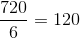.

### Example Question #4 : How To Find An Angle In A Hexagon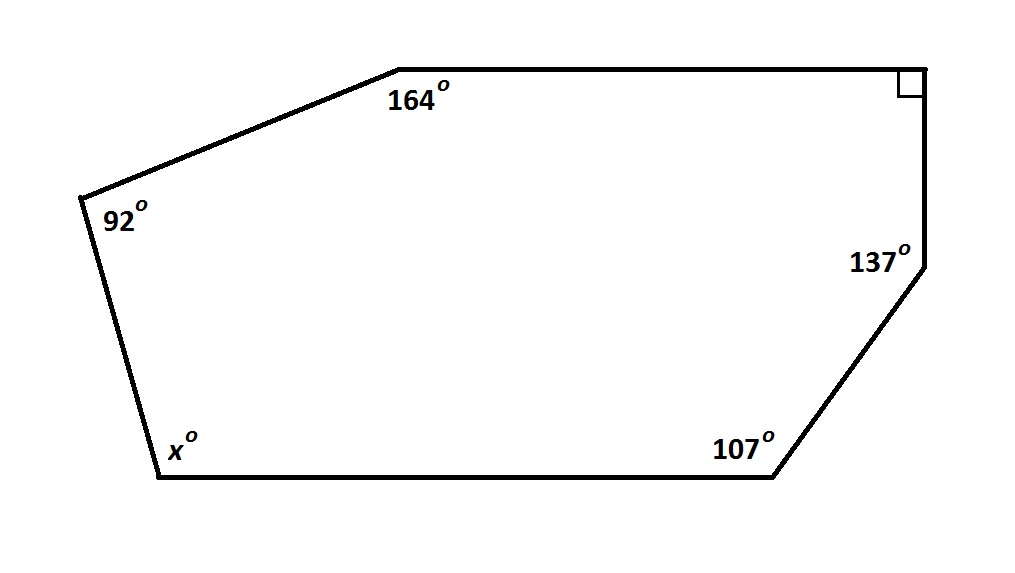Note:Figure NOT drawn to scale.

Refer to the above figure. Evaluate.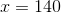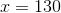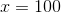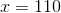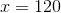Explanation:

The sum of the degree measures of the angles of a (six-sided) hexagon, is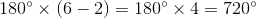We can solve forin the equation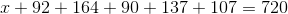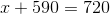### Example Question #5 : How To Find An Angle In A Hexagon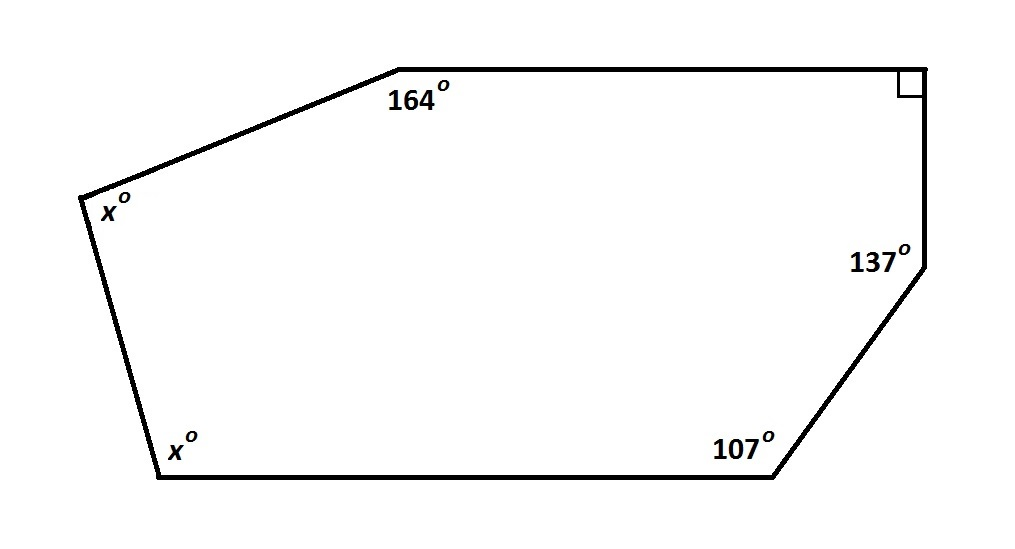Note: Figure NOT drawn to scale.

Refer to the above figure. Evaluate.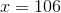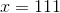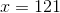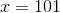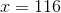Explanation:

The sum of the degree measures of the angles of a (six-sided) hexagon, isWe can solve forin the equation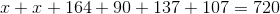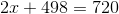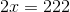### Example Question #6 : How To Find An Angle In A Hexagon

Three angles of a hexagon measure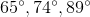. The other three angles are congruent to one another. What is the measure of each of the latter three angles?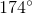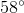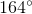This hexagon cannot exist.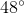Explanation:

The sum of the degree measures of the angles of a (six-sided) hexagon, isLetbe the common measure of the three congruent angles in question. We can solve forin the equation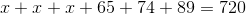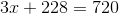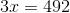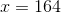### Example Question #7 : How To Find An Angle In A Hexagon

What is the measurement of one of the interior angles of a regular hexagon?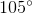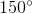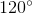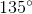Explanation:

To find the sum of the interior angles of any regular polygon, use the formula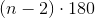, whererepresents the number of sides of the regular polygon.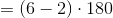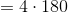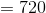The sum of the interior angles of a regular hexagon is 720 degrees. To find the measurement of one angle, divide by the number of interior angles (or sides):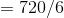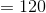The measurement of one angle in a regular hexagon is 120 degrees.